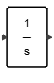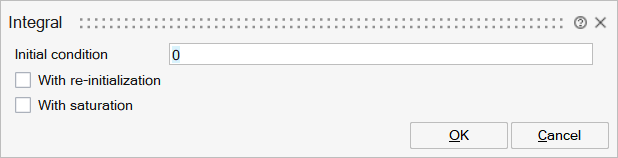# Integral

This block implements an integrator. The output is the integral of the input signal.Dynamical

## Description

The dynamics of the integral block is defined by

dx/dt = u

y = x , x(t0)=x0,

where u, x, y, and x0 are the block input, block state, block output and block initial state values respectively.

dx/dt is the state derivative with respect to time.

Input data type can be double or complex and input can be scalar, vector or matrix.

Example: Bouncing ball

Consider the bouncing ball example with the following modelwhenever the ball hits the ground the ball speed changes as follows:As shown in the figure, two integral blocks are used to model the bouncing ball, one for the h and one for v. The state size pf the model is two and integral blocks are initialized to h0 and 0.0 respectively. A zero crossing block has been used to trigger an event whenever the value of h becomes negative. At the event time the second integrator (v) is reset to new values defined by -0.9*V(hit).

More complex examples are given in the Activate Documentation, such as the Kalmen filter example section.

## ParametersNameLabelDescriptionData TypeValid Values

x0

Initial condition

Defines the initial state of the integrator, which can be a real or complex matrix. If the initial value is scalar and the input is not scalar (vector or matrix), the initial value is expanded automatically to match the internal state size.

Matrix

reinit

With re-initialization

Sets the state to the specified initial condition based on an external signal. If the new state is a function of the previous state (the value just before the reinitialization event), a Lastinput block connected to the integral block output may be used.

Number

0
1

satur

With saturation

Limits the states to a value between the Lower saturation limit and Upper saturation limit parameters.

Number

0
1

limit

Saturation limit

Structure

limit/max

Upper limit

Real value and is active only if the With Saturation parameter is active. If active, the state value will not exceed this limit.

Cell of matrices

limit/min

Lower limit

Real value and is active only if the With Saturation parameter is active. If active, the state value will not be lower than this limit.

Cell of matrices

limit/zcross

Enable zero-crossing

Detects the zero crossings. Default is Off. Active only if the With Saturation parameter is active. Helps to get more precise values at discontinuities.

Cell of numbers

0
1

saturationstatus

Saturation status

If selected, a second output indicates if the integrator is saturated. The output is 1 if the integrator output reaches the upper limit. The output is -1 if the integrator output reaches the lower limit. The output is 0 if the integrator output is not saturated.

Number

0
1

## Ports

NameTypeDescriptionIO TypeNumber

Port 1

explicit

output

1+saturationstatus*satur

Port 2

explicit

input

1

Port 3

explicit

input

reinit

Port 4

activation

input

reinit

NameValueDescription

always active

yes

direct-feedthrough

yes

The feedthrough property of the second input (if present) is always true.

zero-crossing

yes

When Saturation option is checked.

mode

yes

When Saturation option is checked and the solver is variable-step.

continuous-time state

yes

discrete-time state

no